Games
Problems
Go Pro!

# Mental Math

Lesson Plans > Mathematics > Mental Math

# Featured Lesson Plans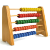A lesson that involves teaching students ways of using algebra to help them do arithmetic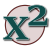Teach algebra students (or younger students) a game that will help them factoring quadraticsVedic Mathematics in a nutshell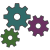To remember Multiplication Table, consider the sum of multiplicand and multiplier.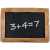There are methods, which are totally unconventional but help in Carrying out tedious and cumbersome arithmetical operations, easily, speedily and in...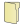# Full Directory Listing

Arithmetic without Calculators, The Sum and Product GameLike us on Facebook to get updates about new resources